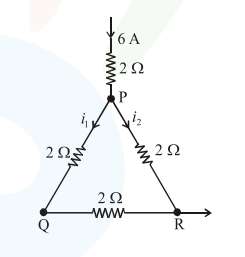# A current of 6 A enters one corner P of an equilateralQuestion:

A current of 6 A enters one corner $P$ of an equilateral triangle $P Q R$ having 3 wires of resistance $2 \Omega$ each and leaves by the corner $R$. The currents $i_{1}$ in ampere is_________.Solution:

For parallel combination current devides in the inverse ratio of resistance.

$\mathrm{i}_{\mathrm{PQ}}=\frac{2}{6} \times 6 \mathrm{~A}$